Next: Problem Up: Gravitational Instability Previous: Linear Analysis   Contents

## Sound Wave

If the self-gravity is ignorable, equations(2.35)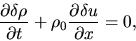(2.40)

and equations(2.37)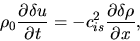(2.41)

where we assumed the gas is isothermal, these two equations describe the propagation and growth of perturbations. If the gas acts adiabatically, replace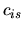with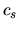.

Making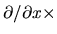(2.40) and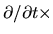(2.41) vanishes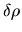and we obtain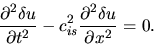(2.42)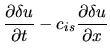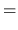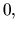(2.43)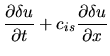(2.44)
equation (2.42) has a solution that a wave propagates with a phase velocity of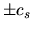. Since the displacement (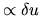) is parallel to the propagation direction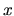, and the restoring force comes from the pressure, this seems the sound wave.